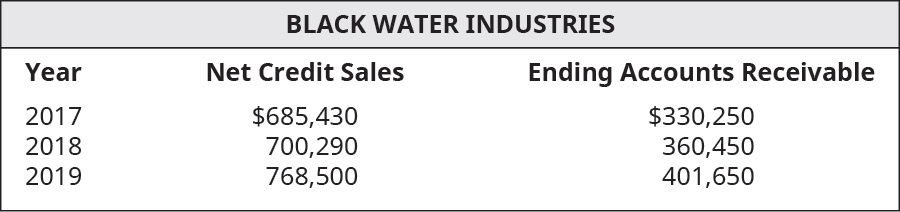# Using the following select financial statement information from Black Water Industries, compute the number of days’ sales in receivables ratios for 2018 and 2019 (round answers to two decimal places). What do the outcomes tell a potential investor about Black Water Industries?FindFindarrow_forward

### Principles of Accounting Volume 1

19th Edition
OpenStax
Publisher: OpenStax College
ISBN: 9781947172685

#### Solutions

Chapter
SectionFindFindarrow_forward

### Principles of Accounting Volume 1

19th Edition
OpenStax
Publisher: OpenStax College
ISBN: 9781947172685
Chapter 9, Problem 9EA
Textbook Problem
36 views

## Using the following select financial statement information from Black Water Industries, compute the number of days’ sales in receivables ratios for 2018 and 2019 (round answers to two decimal places). What do the outcomes tell a potential investor about Black Water Industries?To determine

Concept introduction:

Accounts receivable turnover ratio:

This is an efficiency ratio that indicates the conversion of accounts receivable into cash. This ratio is calculated by dividing the Net credit Sales by the Average accounts receivable. The formula to calculate this ratio is as follows:

Accounts receivable turnover ratio = Net credit SalesAverage accounts receivable

Days Sales uncollected:

This isan efficiency ratio that indicates the period for which credit sales remain as receivable. The ratio is calculated by multiplying Accounts receivable with 365 days and dividing the result by Net Sales. The formula for Days Sales uncollected is as follows:

Days Sales uncollected = Accounts Receivable × 365Net Sales

To calculate:

The Accounts receivable turnover ratio and Days sales in receivable for each year and indicate the outcome to potential investors.

### Explanation of Solution

The Accounts receivable turnover ratio and Days sales in receivable for each year are calculated as follows:

 Year 2018 Year 2019 Net Credit Sales (A) $700,290$ 765,800 Beginning Accounts Receivable (B) $330,250$ 360,450 Ending Accounts Receivable (C) $360,450$ 401,650 Average Accounts Receivables (D)...

### Still sussing out bartleby?

Check out a sample textbook solution.

See a sample solution

#### The Solution to Your Study Problems

Bartleby provides explanations to thousands of textbook problems written by our experts, many with advanced degrees!

Get Started